A 6 minute read

Ancient mathematicians introduced the concept of lines to represent straight objects which had negligible width and depth. Considered as a breadth less length by Euclid, lines form the basis of Euclidean geometry.

When two rays(part of a straight line) intersect each other in the same plane, they form an angle. The point of intersection is called a vertex.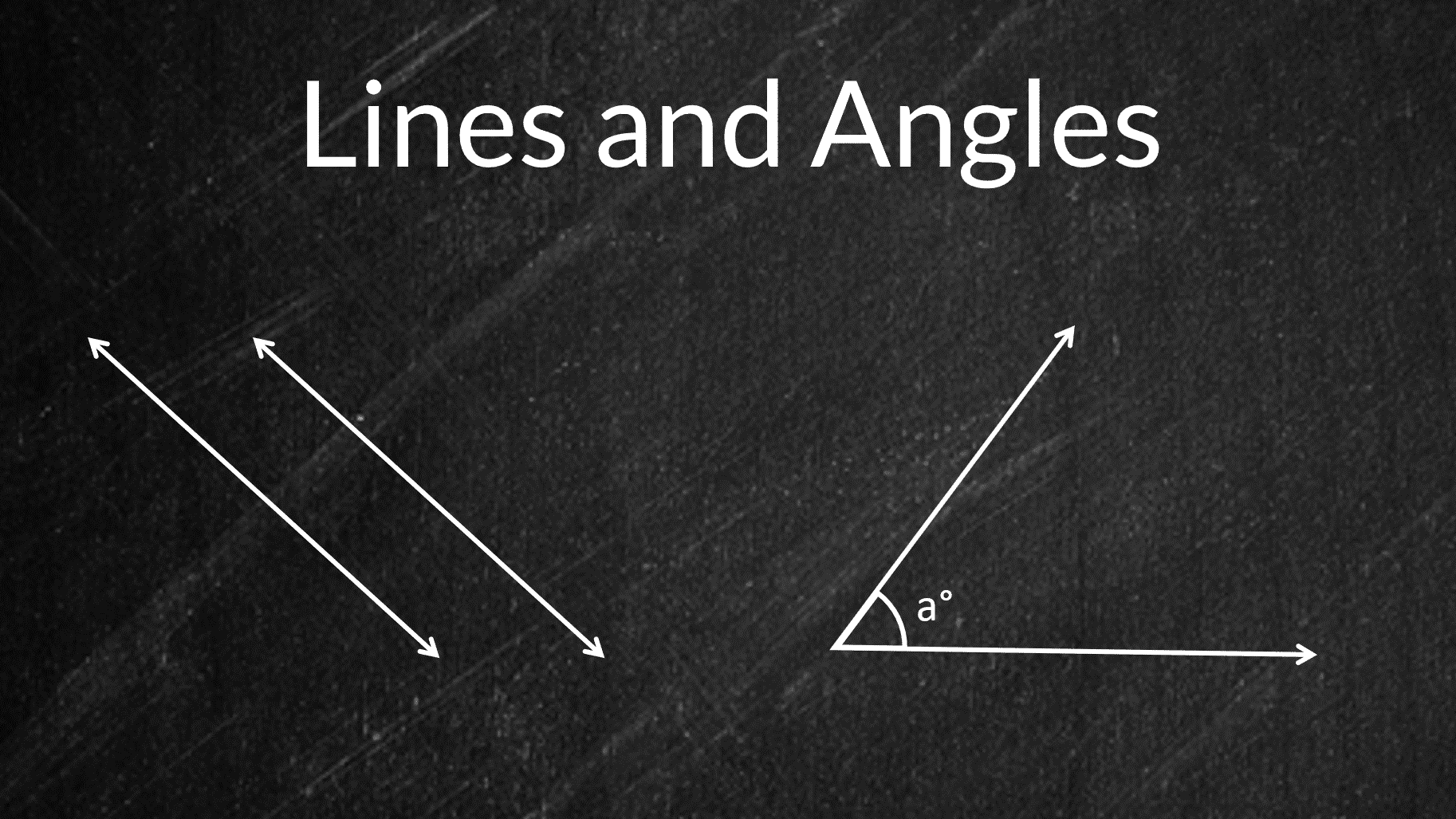Following is a brief outline of the topics that we will cover in this article

## Definition of Line

A line does not have any endpoint.

• It has an infinite length.

## Definition of a Line Segment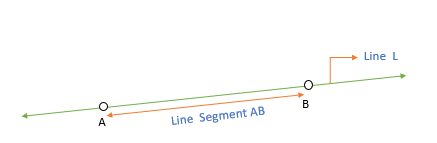A line segment is a segment of a line, or in other words, we can say that a line segment is a line with two endpoints.

For example: The diagram shows a line L and one segment of this line is AB.

In a plane, there can be many lines or line segments.

And, these lines can be divided into a few types based on the relative positioning of a line with another line.

## Types of lines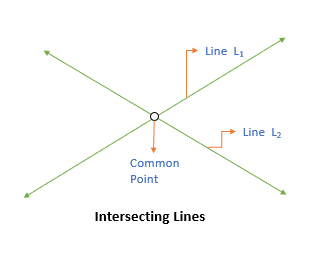### Intersecting Lines

Two lines are intersecting lines if they meet each other at a common point.

For example: L1 and L2 are intersecting lines in the below diagram

### Parallel Lines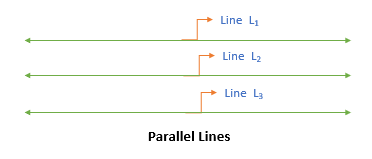A pair of lines are parallel if they never intersect.

For example: L1, L2, and L3 are parallel lines in the below diagram.

### Transversal LineA transversal line cuts two or more lines at distinct points.

For example: Line L3 is the transversal line in the below diagram.

We have now discussed the basics of lines. Let us now discuss angles.

# Angle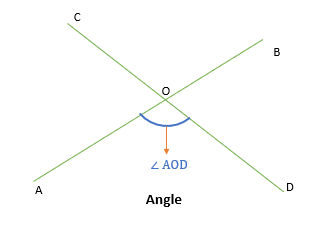An angle is formed when two lines intersect each other. We represent an angle by the symbol ∠.

An angle involves two legs and one common vertex at which two lines meet.

For example: ∠AOD is formed when line AB and CD intersect with each other.

Also, ∠AOD is formed between the leg AO and OD, so we include A, O, and D while naming the angle.

Do you know that the position of leg points does not matter as long as the common vertex is the middle letter in the angle name

## Measurement of an Angle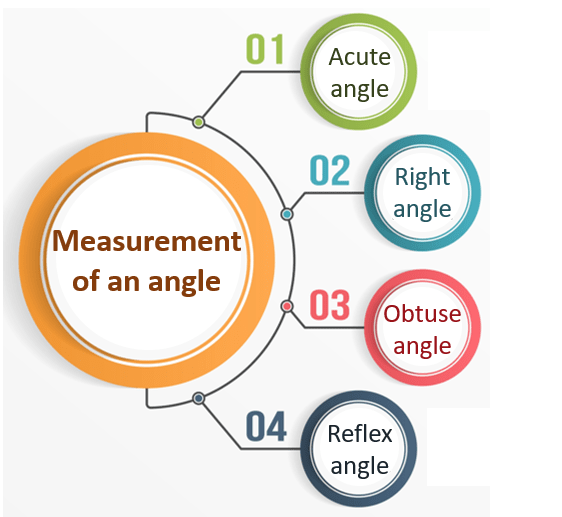The angle is measured in degrees.

An angle can measure from zero (0) degree to 360 degrees.

And, based on the measurement of an angle, they are divided into four types.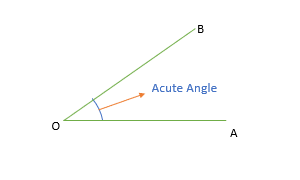### 1. Acute Angle

When the measurement of the angle is between 0 degrees and 90 degrees.

### 2. Right AngleWhen the measurement of the angle is exactly 90 degree.

• If there is a right angle between two lines, then the two lines are said to be perpendicular to each other.

###3. Obtuse Angle

When the measurement of the angle is between 90 degrees and 180 degrees.

A straight line has an angle of 180 degrees.

### 4. Reflex Angle

When the measurement of the angle is between 180 degrees and 360 degrees.

We have discussed the basic type of angles.

Let us now discuss the angles formed when two lines intersect each other.

# 1) Angles formed between two intersecting lines

## 1.1) Vertically Opposite Angles

When two lines intersect each other, then 4 angles are formed.

• And, the angles that are opposite to each other at the intersection point are known as vertically opposite angles.
• Vertically opposite angles are always equal.Let us now discuss the angles formed when two lines are intersected by a third line i.e. a transversal line.

## 2) Angles formed by a transversal lineWhen a transversal line intersects two lines, then eight angles are formed as shown.Now, there are several special pair of angles that are obtained from this diagram.

For example: If you notice  (∠1, ∠3), (∠2, ∠4), (∠5, ∠7), and (∠6, ∠8) are all vertically opposite angles.

Similarly, we get several other types of angles. Let us discuss them.

### 2.1) Interior and Exterior Angles

Interior angles are the ones that are present inside the region between two lines.

• And, exterior angles are the ones that are not present inside this region.

For example:

• ∠2, ∠3, ∠5, and ∠8 are interior angles.
• And, ∠1, ∠4, ∠6, and ∠7 are exterior angles.

### 2.2) Corresponding AnglesTwo angles are said to be corresponding angles if they lie on the same side of the transversal line such that:

• One angle is an interior angle, and
• Another is an exterior angle

For example:

(∠4, ∠8), (∠3, ∠7), (∠1, ∠5), and (∠2, ∠6) are 4 pairs of corresponding angles

### 2.3) Alternate interior angles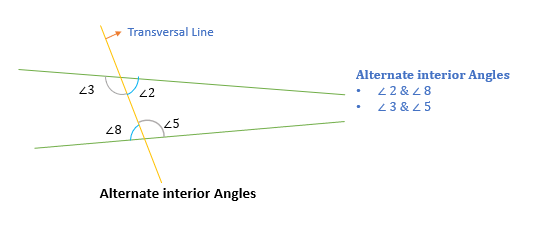Two interior angles, present on the opposite side of a transversal line, are called alternate interior angles.

For example:

• (∠2, ∠8) and (∠3, ∠5) are alternate interior angles.

### 2.4) Alternate exterior angles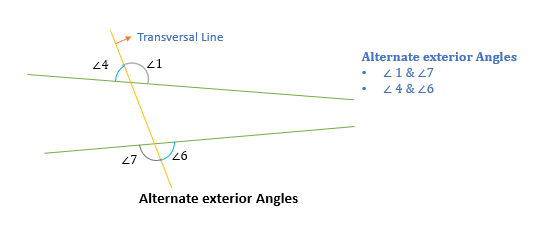Two exterior angles that are present on the opposite side of the transversal line are called alternate exterior angles.

For example:

• (∠1, ∠7) and (∠4, ∠6) are alternate exterior angles.

Note: If a transversal line intersects two parallel lines, then the corresponding angles, alternate interior angles, and alternate exterior angles are equal.

So, we have discussed all the type of angles.

Let us now learn about a few properties of angles.

## Properties of Angles

### 1. Sum of angles on one side of a straight lineSum of all the angles on one side of a straight line is always 180 degree.

For example: The sum of ∠1, ∠2, and ∠3 is 180 degree.

### 2. Sum of angles around a pointSum of all the angles around a point is always 360 degree.

For example: Sum of angles (∠1, ∠2, and ∠3) around point O is 360 degree.

If you liked this article, here are a few more articles that you may like:

## Lines and Angles – Question 1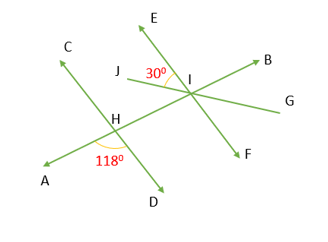In the diagram given below, the line CD is parallel to line EF. If ∠AHD and ∠JIE are 118° and 30° respectively, then what is the measure of angle GIB?

1. 30
2. 32
3. 34
4. 40
5. 62

### Solution

Step 1: Given

• line CD is parallel to line EF.
• ∠AHD =118°
• ∠JIE = 30°

Step 2: To find

• Measure of angle ∠GIB

Step 3: Approach and Working out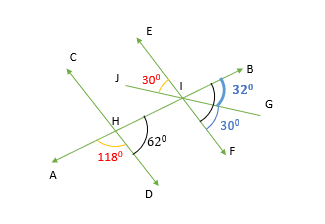We need to find the measure of angle GIB.

• So, with the given information, we know that line CD is parallel to line EF and line AB is transversal to them.
• Hence, ∠DHI = ∠FIB as both are corresponding angles.
• We can find ∠DHI or ∠FIB as
• ∠AHD + ∠DHI = 180°
• 118° + ∠FIB = 180°
• ∠FIB = 62°
• Now, ∠FIB = ∠FIG + ∠GIB
• By observing the diagram, ∠FIG = ∠JIE as these are vertically opposite angles between EF and JG.
• ∠FIB = ∠FIG + ∠GIB
• 62° = ∠JIE + ∠GIB
• 62° = 30° + ∠GIB
• ∠GIB = 32°

Hence, the correct answer is option B.

## Lines and Angles – Question 2In the diagram given below, angle DFE and angle BFC is represented by X and Y respectively. If ∠AFC = 100° and ∠BFE = 45° then what is the value of Y-X?

1. 20
2. 25
3. 30
4. 35
5. 40

### Solution

Step 1: Given

• ∠DFE = X
• ∠BFC = Y
• ∠AFC = 100°
• ∠BFE = 45°

Step 2: To find

• The value of Y-X

Step 3: Approach and Working out

To find Y-X, we need to find Y and X first.

Measure of angle Y:

• We are given ∠AFC = 100° and,
• ∠AFC + ∠BFC = 180° as the sum of angles on the same side straight line is 180°
• 100° + ∠BFC = 180°
• ∠BFC = Y = 80°

Measure of angle X:

• We are given ∠BFE = 45° and,
• ∠DFE + ∠BFE + ∠BFC = 180° as the sum of angles on the same side straight line is 180°.
• X + 45° +80° = 180°
• X = 55°

Hence, Y – X = 80° – 55° =25°.

Thus, the correct answer is option B.Payal Tandon
Co-founder, e-GMAT
Welcome to e-GMAT Support!
I am Payal, Co-Founder of e-GMAT.
Feel free to ask any Query.Thank you for your query.
We will be contacting you soon on